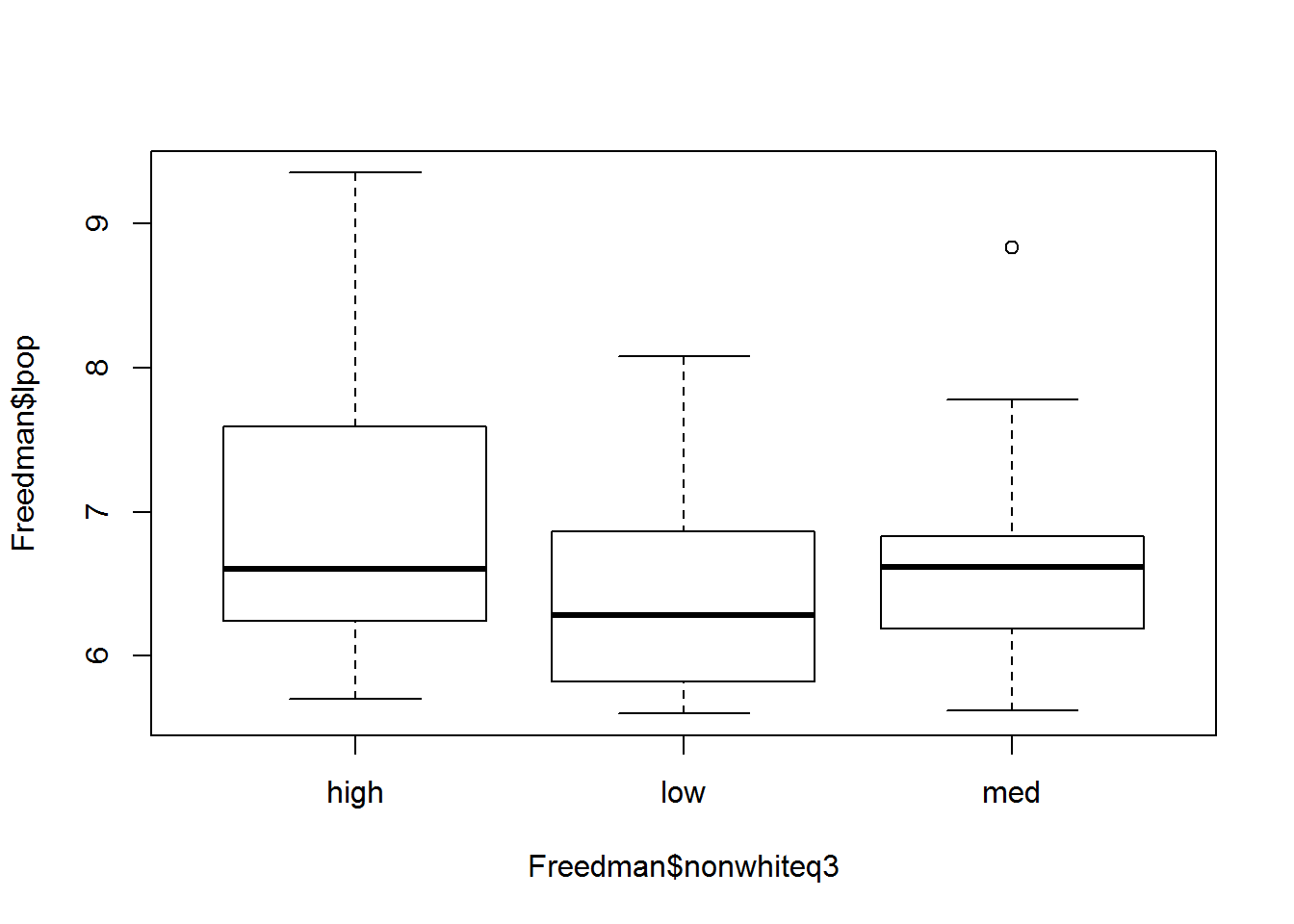This week we will increase the pace of learning by a bit. The goals for this lab are two-fold. First, we will examine the data-frame object and more specifically how to import, read, manipulate, and explore them. Second, we will go over library packages and applied Ordinary Least Squares in R.

# 1. Data frames

Remember vectors and matrices? These were the easy to use R objects that we have been working on during the past few weeks. Data-frames are R objects, but are more complex. Data frames are rectangular data, meaning that they have rows that represent observations and columns representing variables. Unlike programs like SAS, SPSS, and Stata, R has a considerably greater degree of flexibility in manipulating and handling not only rectangular data, but many data frame types. As should be expected though, with greater flexibility comes greater complexity. Let’s go over the data frames by starting with input and import.

## 1.1 input and import

When doing any sort of project in R it is good to keep in mind that there are probably a multitude of ways in which you can code it. Working with data frames is no exception! This lab will not go over the every single way to import, or create, R data frames, but it will go over many of the most useful ways to do so. Let’s start with manual keyboard input.

### 1.1.1 keyboard input

x <- c(1, 2, 3, 4)

x
##  1 2 3 4
names <- c("John", "Sandy", "Mary")

names
##  "John"  "Sandy" "Mary"
v <- c(TRUE, FALSE)

v
##   TRUE FALSE

Remember that we already know a bit about manually inputting data. The above examples show that we can make a variety of different vector objects. For small objects, such as vectors, manually entering data is pretty easy. For larger amounts of data this may be tiresome and error-prone. Let’s try something new.

cooperation <- scan()

#1: 49 64 37 52 68 54
#7: 61 79 64 29
#11: 27 58 52 41 30 40 39
#18: 44 34 44
#21: 

The scan() function allows the user to more easily specify data. Calling scan() will set up a prompt in the command line for you to enter data. Enter the data specified above and create an object called cooperation. Don’t worry about the naming convention right now, but focus on the method. You can end the scan() function call by pressing enter after a blank line.

Another useful function that helps us to create manual data is the rep() function. Let’s try it out here.

rep(5, 3)
##  5 5 5
rep(c(1,2,3), 2)
##  1 2 3 1 2 3

The rep() function, or better known as the replicate function, allows the user to repeat patterns of data. The first argument for rep() is the data that you wish to be repeated, while the second argument specifies the number of times you would like replication to occur. The first example specifies that 5 should be replicated three times. The second example specifies that the vectors (1,2,3) should be replicated twice. Let’s now use rep to make more data for our example data frame.

condition <- rep(c("public", "anonymous"), c(10,10))

condition
##   "public"    "public"    "public"    "public"    "public"
##   "public"    "public"    "public"    "public"    "public"
##  "anonymous" "anonymous" "anonymous" "anonymous" "anonymous"
##  "anonymous" "anonymous" "anonymous" "anonymous" "anonymous"

For the condition variable we simply specifies a character vector that consisted of the words “public” and “anonymous”. The second argument specifies that each character be replicated ten times. Let’s continue with making variables.

sex <- rep(rep(c("male", "female"), c(5, 5)), 2)

sex
##   "male"   "male"   "male"   "male"   "male"   "female" "female"
##   "female" "female" "female" "male"   "male"   "male"   "male"
##  "male"   "female" "female" "female" "female" "female"

You may be confused and that is okay! You may also be thinking, what the hell? Remember that we try to build off each piece of code So lets deconstruct what just happened. You know from the output that we have made a variable called sex that has observations coded as male or female. Notice that we called the rep() function within a rep() function. The inner most function looks like this:

rep(c("male", "female"), c(5,5))

Here we use the rep() function to create a character vector that replicates both male and female five times each.

rep(.....,2)

This outermost rep() function uses the inner rep() code as its target of replication, and orders it to replicate the target twice. This leads us to doubling the five initial replications to ten for each sex specified. This leaves us with the total of twenty observations created!

Now lets put all three vectors (cooperation, condition, sex) into a data frame.

Guyer <- data.frame(cooperation, condition, sex)

We have created our first data frame in R! From a substantive standpoint, you have manually inputted data from a psychology experiment conducted by Fox and Guyer (1978) that examined cooperative behavior and public/anonymous identity.

### 1.1.2 text file import

While the above example illustrates the power of R to easily create data, it is unlikely that you will want to manually create data frames for your projects. Fortunately R has you covered! The first such function for importing data is the read.table() function. This function imports plain text files into R as data frames. Let’s try it out! First, download the job prestige data set by clicking here. Save the text file into your working directory (should be where your project is located.). Saving any data file into your working directory makes file pathing much easier!

prestige <- read.table("prestige.txt", header=TRUE)

view(prestige)

The read.table() function has loaded this data set into R! The read.table() function also works with .csv files as well as .txt files. There is also a function called file.choose() that can be called from within read.table() that make searching for files a bit easier.

prestige <- read.table(file.choose(), header=TRUE)

This will pull up a window for you to manually navigate to the data file of your choice.

A final method of retrieving data files is by scraping them off of the internet. This method skips the download process and automatically loads the data into R. First, clear your objects from working memory to get rid of prestige.

rm(list = ls())

view(prestige)

Wow! That was super easy. Anytime that a data file of interest is housed on the internet, you can call the url as the data source path. One final example concerns how to save this newly imported prestige data set. This can be done with the write.table() function. Lets try it below.

write.table(prestige, "prestige2.txt")

You should now see both a prestige.txt and a prestige2.txt in your working directory window within R Studio.

### 1.1.3 foreign file import

In addition to the text files, R is capable of importing data sets from almost any other statistical package format. This generally requires the use of the foreign package. Lets try installing and loading the foreign library package.

install.packages("foreign")

library(foreign)

The foreign package allows you to import and export spss, SAS, and Stata data files. The logic of the functions provided by the foreign package is the same as the text import/export above. I recommend typing help(foreign), or googling the package to get a better sense of what it can do and what read/write functions are appropriate for you.

Much of the time our data comes in Excel format. An easy way to deal with this is to save your Excel spreadsheet as a .csv file and use the read.table(), but it is possible to directly import Excel files into R. To do this, we can use the xlsx package.

install.packages("xlsx")

library(xlsx)

Again the logic behind the xlsx package functions is functionally the same to read.table(). Use help(xlsx) to get more specific information.

### 1.1.4 data frames from R packages

The final way to access data frames is to call them from a specific library package. Lets download and load the car package and use it to access the prestige data set.

rm(list = ls())

install.packages("car")

library(car)

data(Prestige, package="car")

head(Prestige)

Accessing data from packages will probably be the least used of the above strategies, but it is important to know none the less. Many advanced tutorials utilize data from packages.

## 1.2 working with data frames in R

Now that we know how to import and export data into/from R, we can begin to work with the data frame class. There are two ways for working with data frame object information. The first is to use the attach() function, and the second is to explicitly call data frame object information. Lets try the first method.

attach(Prestige)

search()

The search() shows the global environment and all objects that are stored in working memory. These objects have first priority when calling up data from R code. While this makes accessing objects from Prestige easier, it also has some downsides. If you are working with multiple data frames, and have them attached to memory, than it is possible that your code will only access objects from the data frame that has priority status. This is likely to happen if you have multiple data sets that share variable names.

I always recommend to work with data frames explicitly and to avoid the attach() function. The code below can remove an attached data frame object.

detach(Prestige)

search()

detach() removes the object, and search() confirms that it is gone from working memory.

### 1.2.1 explicitly calling objects from data frames

We can explicitly use the Prestige data frame in a number of ways. Try typing the lines of code below.

summary(Prestige)

mean(Prestige$prestige) mean(Prestige$income)

mean(Prestige$census) lm(prestige ~ income + education, data = Prestige) Most of the above code should at least make some sense to you, even if you do not fully understand yet. The summary() provided summary statistics of each variable housed in the prestige data frame. The mean() functions calculated the mean of each variable called. Take notice of how we called these variables in the above code. In previous examples we were able to use the mean() function on any object, but here we specified Prestige$prestige for the prestige variable. The $operator is the important concept here. The first object “Prestige” is the data frame of interest, and the second object “prestige” is a variable housed within this data frame. The$ operator explicitly calls a variable from a data frame. If you are not attaching a data frame to memory, you MUST always use the $operator to explicitly call a single variable from a data frame. The final line of code is a bit new to you. What do you think this is? (hint: look at the output). The lm() function is the function that estimates an ordinary least squares regression (OLS). Here we explicitly call variables from the Prestige data frame in a slightly different way. The lm() function has an argument called data, which takes the name of the data frame used. ### 1.2.2 missing data R gives us powerful tools for examining and fixing issues with data frames. To illustrate how R can work with missing data lets call another data set from the car package. data(Freedman, package="car") head(Freedman) The head() function simply calls the first six observations of a data set, with six being the default number of observations displayed. You can specify a greater or lesser number of rows to examine, as well as calling the last six observations within a data frame by using the tail() function. head(Freedman, n = 10) # calls first ten rows (observations) tail(Freedman) # calls last six rows (observations) Examining the initial six observations, we can see that density has a value of NA for Albuquerque. NA is R’s way of stating that data is missing. Lets further explore the density variable. head(Freedman$density, 20) #extract first twenty values of density in the freedman data frame

Remember that by using the $operator we are explicitly calling a data frame object (variable). In this case, we use the head() function to extract the first twenty values of density. The output shows that we have at least three missing density values! Lets try looking at central tendency measures for density… mean(Freedman$density)
##  NA
median(Freedman$density) ##  NA When we try to calculate the mean and median of density, the output returns only a NA value. This is because many R math functions are unable to calculate a quantity of interest in the absence of data as a default. To calculate these central tendency measures, we must specify an additional argument to take into account missing observations. mean(Freedman$density, na.rm=TRUE)
##  765.67
median(Freedman$density, na.rm=TRUE) ##  412 After specifying na.rm = TRUE, we finally get numerical output. The na.rm argument simply tells the function to remove all na values from the calculation. The reason we were unable to calculate mean/median the first time is because the na.rm argument is set to FALSE by default. Many other R functions such as var(), sd(), quantile(), etc also utilize na.rm arguments for missing data. Other types of R functions, such as plot() and lm() automatically ignore missing data by default. When using a new function, it is a good idea to use the help() function to see whether it automatically handles missing data or not. A quick and easy way to filter out observations with missing data (list wise deletion) is to use the na.omit() function. Try typing the following code. Freedman.complete <- na.omit(Freedman) The result is a brand new data set that has dropped ten observations with missing data without substantively altering the original data frame object. ### 1.2.3 modifying and transforming variables in a data frame R makes it very easy to transform, or modify, variables within a data frame object. Lets create some mathematical transformations for variables in the Freedman data set. lpop <- log(Freedman$population)

lcrime <- log(Freedman$crime) den2 <- Freedman$density^2

densq <- sqrt(Freedman$density) The above code created four new vector objects in your R Studio environment window. The first two create log transformations, while the third exponentiates density to the second power and the fourth takes the square root of density. But what if we want to add these transformations to our data set as opposed to simply creating new vector objects? Freedman$lpop <- log(Freedman$population) Freedman$lcrime <- log(Freedman$crime) Freedman$den2 <- Freedman$density^2 Freedman$densq <- sqrt(Freedman$density) By using the explicit data frame call (Freedman$newobject), you can store the new vector object as a variable in any data frame you wish! Look at how your Freedman object now has 8 variables instead of 4. Another type of transformation that can be done is to cut a interval level variable into a series of cut-off values. This can be done by using the cut() function.

Freedman$nonwhite3 <- cut(Freedman$nonwhite, 3, labels = c("low", "med", "high"))

summary(Freedman$nonwhite3) Here we transformed the variable nonwhite (percentage of non white population) into three discrete cut off classes. The first argument is the data we wish to cut into bins, the second argument is the number of bins (n = 3 here), and the third argument (labels) specifies the names of the bin labels. This essentially changes it into an ordinal level factor variable. Each factor is of equal numerical width, but observations are not equal among each category. We can try and transform nonwhite into factor categories with greater dispersion by using quantile separation. Freedman$nonwhiteq3 <- cut(Freedman$nonwhite, quantile(Freedman$nonwhite, c(0, 1/3, 2/3, 1)),
include.lowest = TRUE,
labels=c("low", "med", "high"))

summary(Freedman$nonwhiteq3) The logic for the quantile transformation is the same for the first example of cut(), but we add in the include.lowest argument to ensure that all values are used in the quantile transformation. Now lets save the freedman data frame that now contains our six new variables to our working directory. write.table(Freedman, "freedman2.txt") ## extra credit: plot data frame objects. Lets use what we learned about explicitly calling data frame variables by plotting them in different ways. plot(Freedman$population)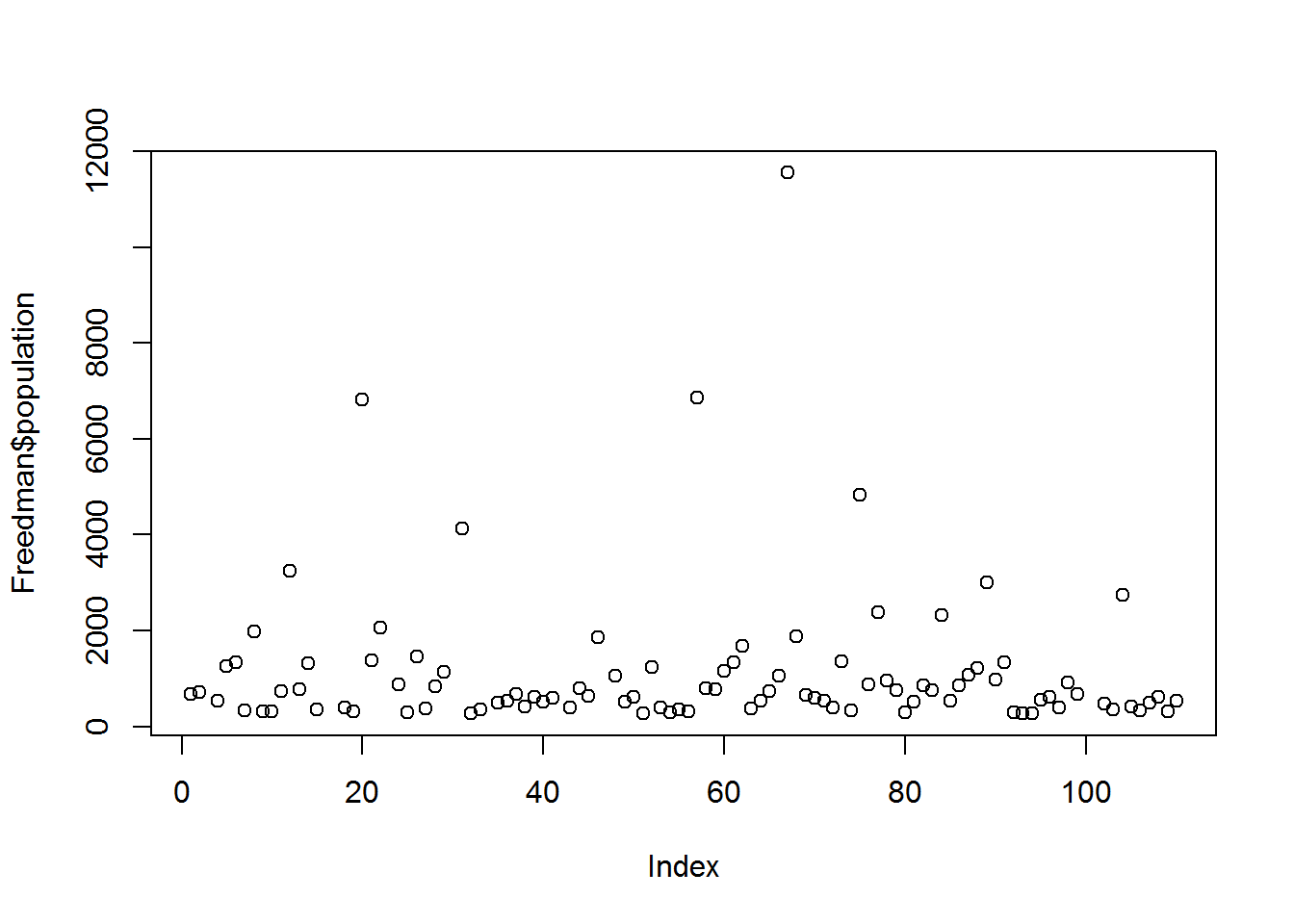plot(Freedman$lpop)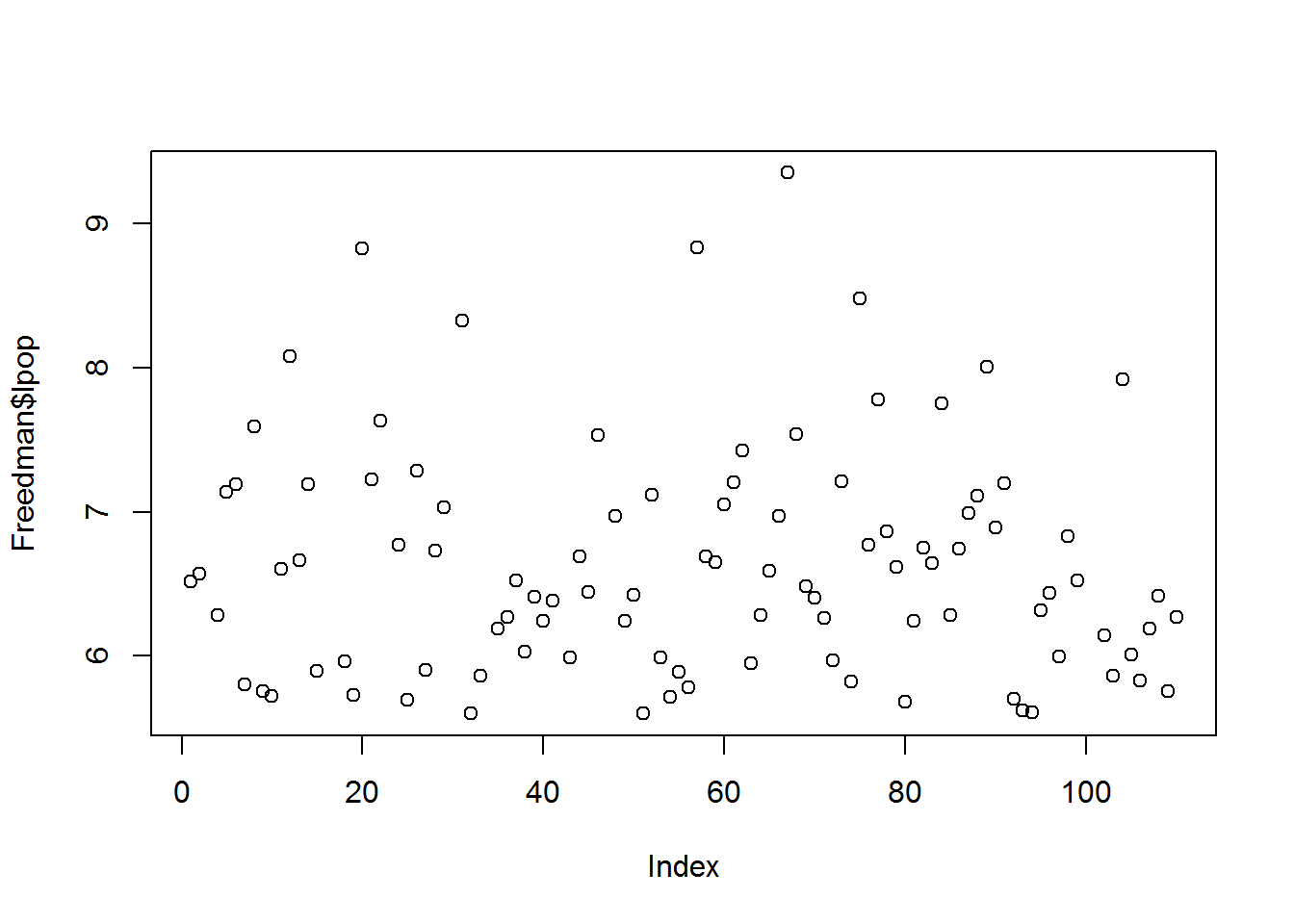plot(Freedman$nonwhite3)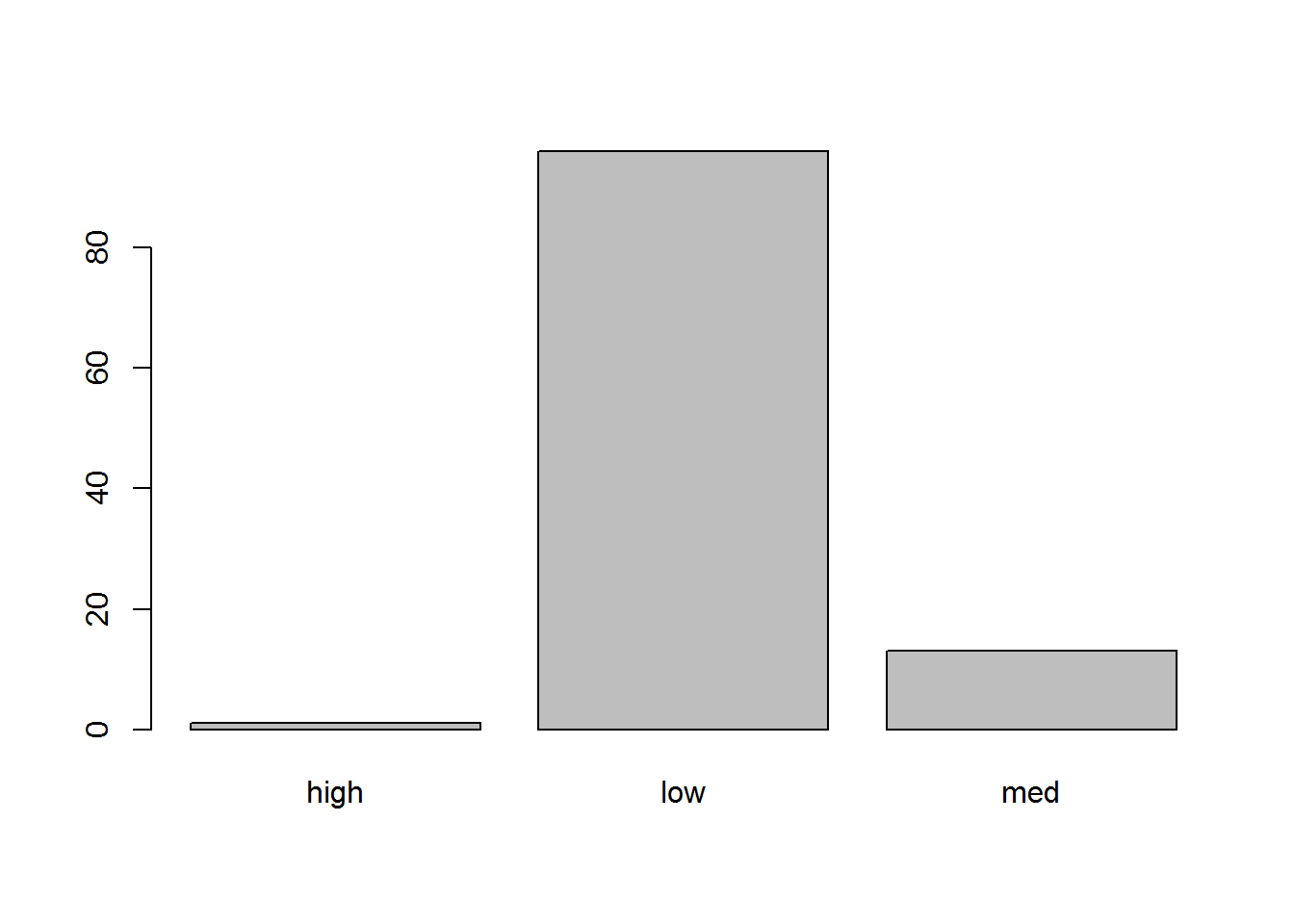plot(Freedman$nonwhiteq3)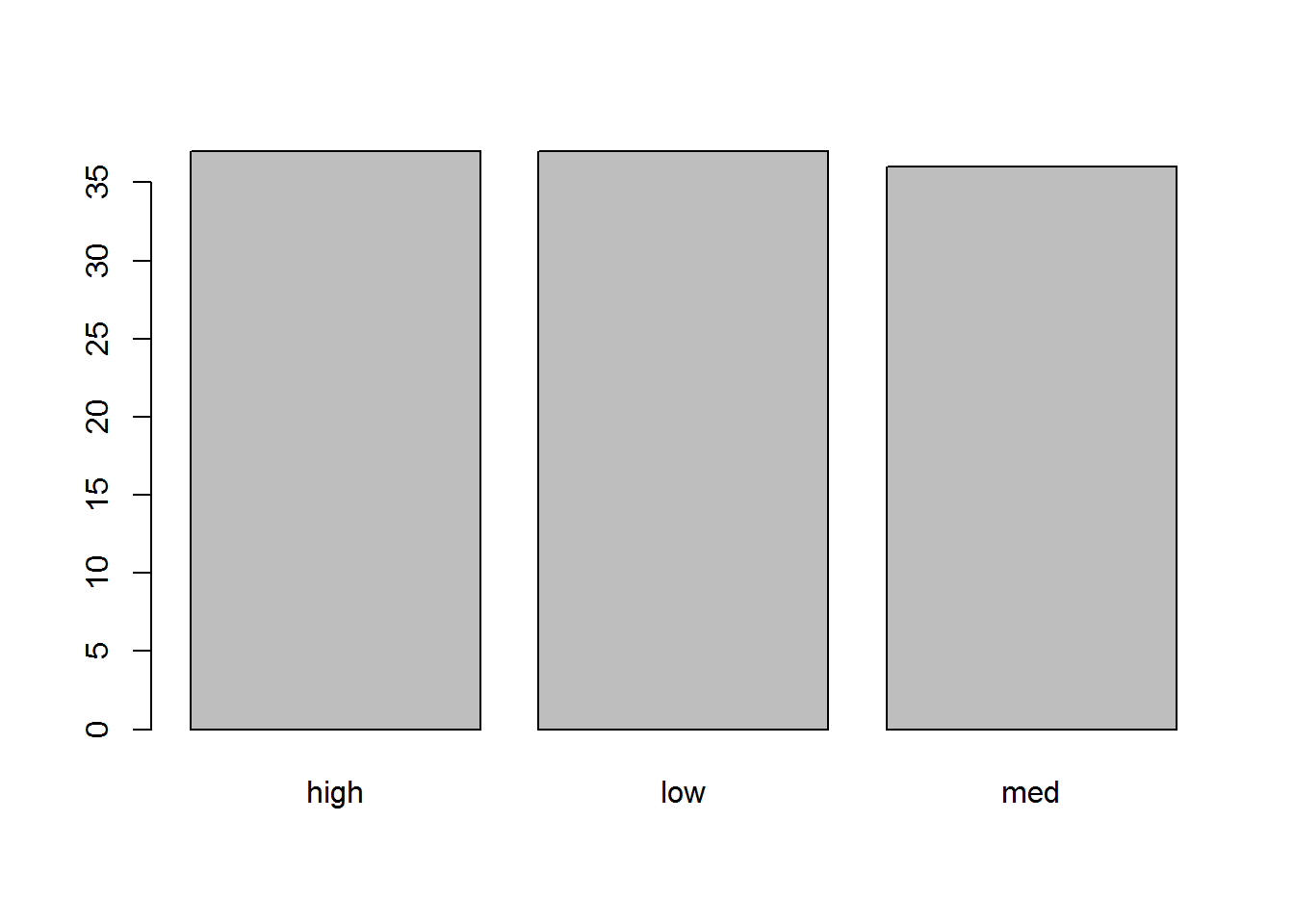plot(Freedman$lpop ~ Freedman\$nonwhiteq3)